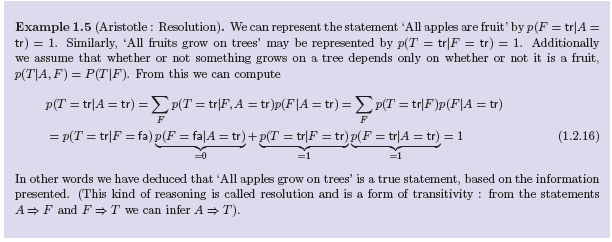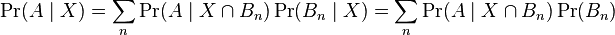# Example involving conditional probability and transitivity

I'm just going to post a screenshot of the Example (free online textbook). I'm having a tough time making the leap to the first sum - what allows me to rewrite P(T|A) as the sum of the product of those two conditional probabilities?Thanks

Office_Shredder
Staff Emeritus
Gold Member
2021 Award
It doesn't have anything to do with the fact that you have a conditional probability to start, it's an application of the more general statement
$$P(X=true) = P(X=true | Y = true)P(Y= true) + P(X = true | Y = false)P(Y = false)$$

Well I understand the bolded statement. I don't know why it doesn't have anything to do with the fact that I'm dealing with a conditional probability, since it's P( T = tr | A = tr ). In my mind I'm looking for an algebraic substitution or something that I know that allows me to manipulate this into something resembling P(T|A,F). What I don't know is where the P(T|A,F)*P(F|A) comes from or how I could get there.

For example, why not P(T|F,A)*P(F) and sum over F? Why is it P(F|A)?

Ok, I found what I was looking for. It's an application of the law of total probability for conditional probabilities:Stephen Tashi